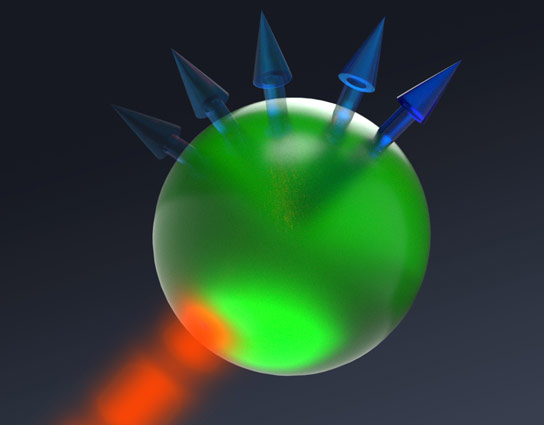# First Silicon Quantum Logic Gates

Written by: Dimitri Leggas
Edited by: Hsin-Pei Toh

The allure of quantum computing has not worn off since mathematicians and physicists like Yuri Manin and Richard Feynman theorized the field in the 1980s. Quantum computing promises rapid speed-ups in computations that can be parallelized, with potential impact on data security, as well as the financial and health industries. Currently, the development of functional quantum computers is at an early stage. However, recent advances made by a team at the University of New South Wales (UNSW) have shown that quantum logic gates can be constructed in silicon, an industry standard material for computer chips and transistors.

The theoretical increase in speed derived from operating a quantum computer stems from the ability to store exponentially more information in a quantum bit, or qubit, than in a standard bit. Modern digital computers are based on series of transistors, which control the flow of electrons to define two possible states for a bit: 0 or 1. Logic gates, systems that take two inputs and return a single output, form the basis of digital circuits. In the quantum arena, qubits are transistors that are reconfigured to hold a single electron, and the spin of this electron determines the state. Unlike bits, a qubit’s state is not one of two definite states, but rather may be in a superposition of states. Suppose one has three qubits. The state of those qubits is a probability distribution of the 23=8 possible states: 000, 100, 010, 001, 110, 101, 011, 111, whereas in a classical computer the state of the bits is precisely one of those options with probability 1. Despite the dramatic increase in information storage capacity, there are inherent drawbacks to storing information in this way. Since the states are non-deterministic, there is no guarantee that an algorithm returns the same answer to a problem each time it is solved. So a process must be continuously repeated in order to determine if the answer found is correct. Additionally, the superposition of states is only beneficial if data can be processed in parallel, meaning that each step does not rely on the previous. If a certain process is mostly sequential, quantum computations are not faster than classical ones.The state of a qubit is not definite, but a superposition of states based on the spin of an electron.

Quantum processes rely on the entanglement of qubits, which until recently had only been produced in expensive materials like diamonds and cesium. Researchers Menno Veldhorst and Andrew Dzurak, researchers at UNSW, have improved existing industry technologies to entangle two qubits in silicon transistors by using microwave fields and voltage signals to force the two qubits to interact with one another, so that the state of second qubit depends on that of the first. In the logic gate built by the UNSW team, there is a target qubit and a control qubit. The target qubit maintains its original spin if the control qubit points upward (value of 1), but changes its spin if the control qubit is pointing downward (value of 0). Veldhorst says that extrapolating from this two-qubit gate will allow any size gate set to be constructed, making an incredible array of quantum computations possible. In addition to the development of the two-qubit gate, the UNSW scientists have developed a method by which the number of qubits can be scaled to millions, leading to the development of the first quantum computer chip. Despite these significant developments, one serious drawback remains. The quantum logic gate built at UNSW cannot function at room temperature, since it must be cooled to 1 Kelvin (or -458 degrees Fahrenheit). Nonetheless, Veldhorst and Dzurak remain optimistic because of recent progress made in cooling and refrigeration.

The possibility, or perhaps inevitability, of quantum computers is promising. However, quantum computing will change how humans approach problems in fields like cryptography. For example, the Rivest-Shamir-Adleman (RSA) cryptosystem, a cryptosystem for public-key encryption, is highly dependent on the long time duration required to factor large numbers into primes. In RSA, a public key consisting of two large primes is used to encrypt data, and a private key is used to decrypt the data. If one does not know the private key, then they would have to factor a large number to decrypt the data, which currently is a practical impossibility. But with the powerful parallel potential to be found in quantum processors and Shor’s algorithm, a quantum algorithm for factoring numbers using parallel processes, potential hackers could easily render RSA useless.

This small example in cryptography reflects the incredible impact of quantum computing on our daily lives. While quantum computers may not be necessary for the everyday consumer needs of most individuals, they will nonetheless change underlying processes that concern our every decision, in particular when using the internet.